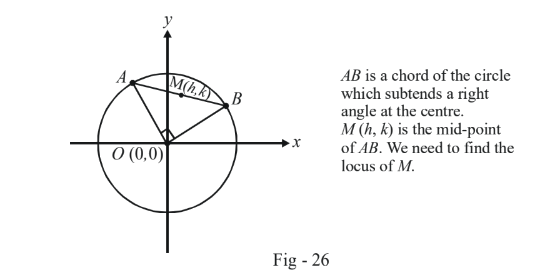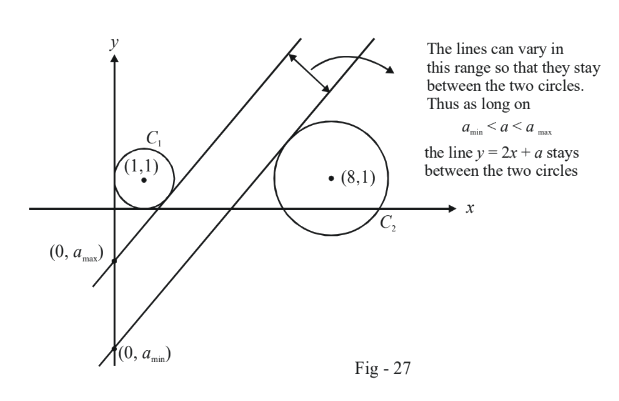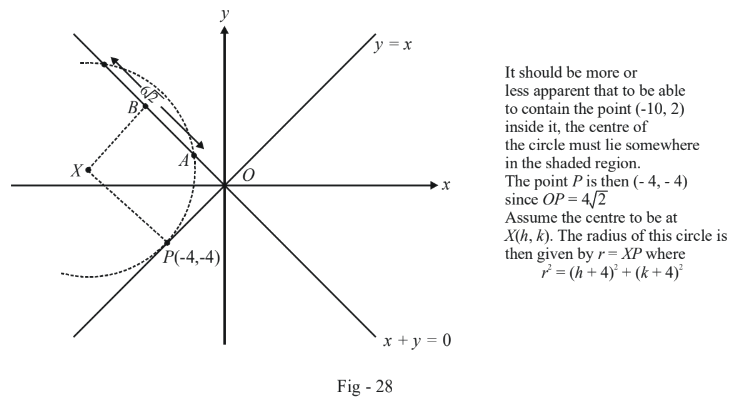# Examples On Tangents To Circles Set-4

Go back to  'Circles'

Example - 26

Find the locus of the mid-point of the chords of the circle $${x^2} + {y^2} = {a^2}$$ which subtend a right angle at the centre.

Solution:Since $$AB$$ is bisected at $$M(h,k),$$ we can use the result obtained in the last example to write the equation of $$AB$$:$\begin{array}{l}\,\,\,\,\,\,\,\,\,\,\,\,\,\,\,\,&T(h,k) = S(h,k)\\ \Rightarrow & hx + ky - {a^2} = {h^2} + {k^2} - {a^2}\\ \Rightarrow & hx + ky = {h^2} + {k^2}\end{array}$

We can view the chord $$AB$$ as a line intersecting the curve (circle) $${x^2} + {y^2} = {a^2}.$$  Thus, we can obtain the joint equation of $$OA$$ and $$OB$$ by homogenizing the equation of the circle using the equation of the chord AB:

\begin{align}{x^2} + {y^2} - {a^2}{\left( {\frac{{hx + ky}}{{{h^2} + {k^2}}}} \right)^2} = 0\end{align}

Joint equation of

OA and OB:

$\Rightarrow \qquad {({h^2} + {k^2})^2}({x^2} + {y^2}) - {a^2}{(hx + ky)^2} = 0\,\,\,\,\,\,\,\,\,\,\,\,\,\,\,\,\,\,\,\,\,\,\,\,\,...(1)$

Since $$OA$$ and $$OB$$ are perpendicular, we must have

${\text{Coeff }}{\text{.of }}{x^2} + {\text{Coeff.}}{\text{ of }}{y^2} = 0$

$\Rightarrow \qquad 2{({h^2} + {k^2})^2} - {a^2}{h^2} - {a^2}{k^2} = 0$

$\Rightarrow \qquad 2{({h^2} + {k^2})^2} - {a^2}{h^2} - {a^2}{k^2} = 0$

Using $$(x,y)$$ instead of$$(h,k),$$ we obtain the following equation as the locus of $$M$$:

${x^2} + {y^2} = \frac{{{a^2}}}{2}$

This is a circle concentric with the original circle as might have been expected.

Try solving this question using pure geometric considerations; the solution will be much simpler.

Example - 27

Consider two circles with the following equations:

$\begin{array}{l}{C_1}:\,\,\,\,\,{x^2} + {y^2} - 2x - 2y + 1 = 0\\{C_2}:\,\,\,\,\,{x^2} + {y^2} - 16x - 2y + 61 = 0\end{array}$

Find the values that a can take so that the variable line $$y = 2x + a$$ lies between these two circles without touching or intersecting either of them.

Solution: Observe carefully that what is variable about the variable line $$y = 2x + a$$ is not its slope but its   $$y$$-intercept $$a$$. Thus, we can always adjust $$a$$ so that line stays between the two circles. The following diagram makes this clear.Evaluate $$\;{a_{\max }}$$ : The line $$y = 2x + {a_{\max }}$$ is a tangent to $${C_1}$$ if the perpendicular distance of the centre $$(1, 1)$$ of $${C_1}$$  from this line is equal to $$C_1^{\,'}s$$ radius which is $$1$$. Thus :

\begin{align}{}\,\,\,\,\,\,\,\,\,\,\,\,\,\,\,\,\,&\frac{{\left| {2 - 1 + {a_{\max }}} \right|}}{{\sqrt 5 }} = 1\\ \Rightarrow \qquad & 1 + {a_{\max }} = \pm \sqrt 5 \\ \Rightarrow \qquad & {a_{\max }} = - \sqrt 5 - 1\,\,\,\,\,\,\,\,\,\,\,\,\,\,\,\,\,\,\,\,\left\{ \begin{array}{l}{\text{since from the figure we }}\\{\text{can see that }}{a_{\max }}{\text{is definitely}}\\{\text{negative}}\end{array} \right\}\end{align}

Evaluate $${a_{\min }}$$ :   The distance of $$C_2^{\,'}s$$ center $$(8, 1)$$ from $$y = 2x + {a_{\min }}$$ must be equal to its radius which is equal to $$2$$. Thus :

\begin{align}{}\,\,\,\,\,\,\,\,\,\,\,\,\,\,\,\,\,&\frac{{\left| {16 - 1 + {a_{\min }}} \right|}}{{\sqrt 5 }} = 2\\ \Rightarrow & 15 + {a_{\min }} = \pm 2\sqrt 5 \\ \Rightarrow & {a_{\min }} = 2\sqrt 5 - 15\,\,\,\,\,\,\,\,\,\,\,\,\,\,\,\,\,\,\,\,\,\,\,\,\,\,\,\,\,\,\,\,\left\{ \begin{array}{l}{\text{we have selected the larger of the two}}\\{\text{values possible since that is what }}\\{\text{corresponds to }}{a_{\min }},{\text{i}}{\rm{.e}}{\text{. because the}}\\{\text{line }}y = 2x + {a_{\min }}{\text{ lies above }}{\text{ }}{C_2}.\end{array} \right\}\end{align}

Thus, we obtain the allowed values of a as

$2\sqrt 5 - 15 < a < - \sqrt 5 - 1$

Example - 28

A circle touches the line $$y = x$$ at a point $$P$$ such that $$OP = 4\sqrt 2$$ where $$O$$ is the origin. The circle contains the point $$(–10, 2)$$ in its interior and the length of its chord on the line $$x + y = 0$$ is $$6\sqrt 2 .$$ Determine the equation of the circle.

Solution: As always, before starting with the solution, it is a good to draw a diagram of the situation described to get a feel of it. Also, as far as possible, we should try to use pure-geometric considerations to cut down on the (complicated) algebraic manipulations that would result otherwise.We have,

\begin{align}{}\,\,\,\,\,\,\,\,\,\,\,\,\,\,\,&PX \bot (y = x)\\ \Rightarrow \qquad & \frac{{k + 4}}{{h + 4}} = - 1\,\,\,\,\,\,\,\,\,\,\,\,\,\,\,\,\,\,\,\,\,\,\,\,\,\,...(1)\\ \Rightarrow \qquad & h + k + 8 = 0\,\,\,\,\,\,\,\,\,\,\,\,\,\,\,\,\,\,\,\,\,\,\,...(2)\end{align}

Also,

\begin{align}&\left\{ \begin{array}{l}{\rm{Perpendicular dist}}{\rm{.}}\\{\rm{of }}X{\rm{ from }}x + y = 0\end{array} \right\} = BX = \sqrt {A{X^2} - A{B^2}} = \sqrt {{r^2} - A{B^2}} \\ \Rightarrow \qquad & \frac{{\left| {h + k} \right|}}{{\sqrt 2 }} = \sqrt {{{(h + 4)}^2} + {{(k + 4)}^2} - {{(3\sqrt 2 )}^2}} \,\,\,\,\,\,\,\,\,\,\,\,\,\,\,\,\,\,\,\,\,\,\,\,\,\,\,\,...(3)\end{align}

Using (1) and (2) in (3), we obtain

\begin{align}&{\left( {4\sqrt 2 } \right)^2} = 2{\left( {h + 4} \right)^2} - 18\\ \Rightarrow \qquad & h + 4 = \pm 5\\ \Rightarrow \qquad & h = - 9,1\end{align}

Given the region in which $$X$$ lies, $$h$$ must be $$–9$$. Thus, from (2), $$k$$ is $$1$$ and the radius $$r$$ is $$5\sqrt 2$$ .

The required equation is therefore

\begin{align}\,\,\,\,\,\,\,\,\,\,\,\,\,\,\,\,\,&{(x + 9)^2} + {(y - 1)^2} = {(5\sqrt 2 )^2}\\ \Rightarrow \qquad & {x^2} + {y^2} + 18x - 2y + 32 = 0\,\,\,\,\,\,\,\,\,\,\,\,\,\,\,\,...\,(4)\end{align}

For students used to rigor, it can finally be verified that the circle given by (4) does indeed contain the point $$(-10, 2)$$ and thus our initial assumption of the region in which the centre $$X$$ lies, was correct.